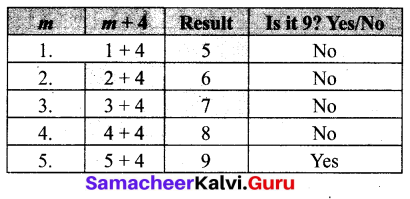# Samacheer Kalvi 6th Maths Solutions Term 1 Chapter 2 Introduction to Algebra Intext Questions

You can Download Samacheer Kalvi 6th Maths Book Solutions Guide Pdf, Tamilnadu State Board help you to revise the complete Syllabus and score more marks in your examinations.

## Tamilnadu Samacheer Kalvi 6th Maths Solutions Term 1 Chapter 2 Introduction to Algebra Intext QuestionsTry These (Text hook Page No. 41)

Question 1.
Observe the following patterns and complete them.
(i) 5, 8, 11, 14, ___, ____, ____
(ii) If 15873 × 7 = 111111 and 15873 × 14 = 222222 then, what is 15873 × 21 = ? and 15873 × 28 = ?
Solution:
(i) 17, 20, 23
Hint: 5 + 3 = 8, 8 + 3 = 11, 11+3 = 14
(ii) 15873 × 21 = 333333; 15873 × 28 = 444444
Hint: 15873 × 14 = 15873 × 7 × 2 = 111111 × 2 = 222222

Question 2.
Draw the next two patterns and complete the table.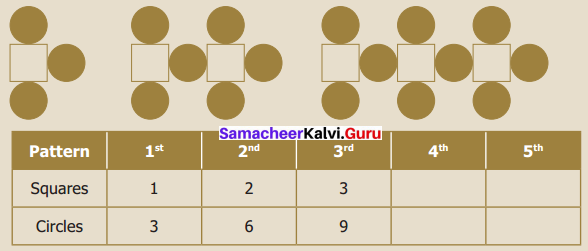Solution:
The next two patterns: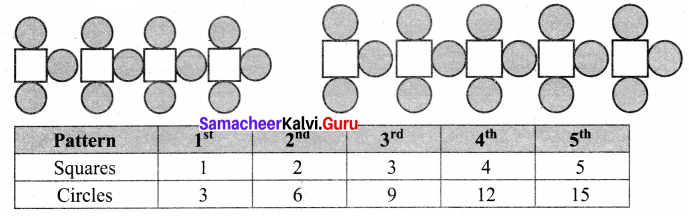Question 3.
Create your own patterns of shapes and prepare a table.
Solution:
(i) Match stick pattern of triangles.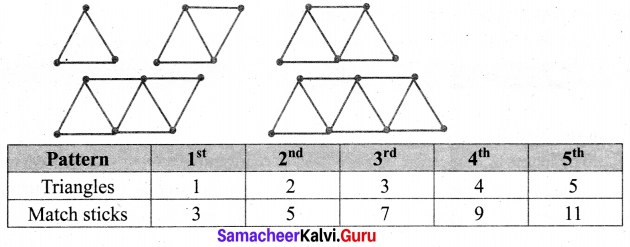(ii) Pattern of squares and circles.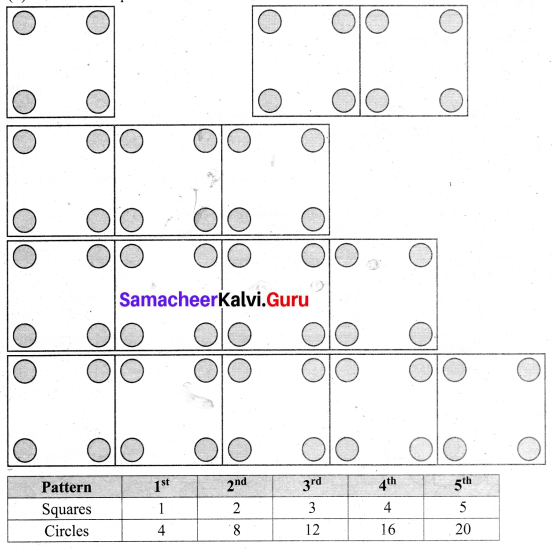Try These (Text hook Page No. 46 to 48)

Question 4.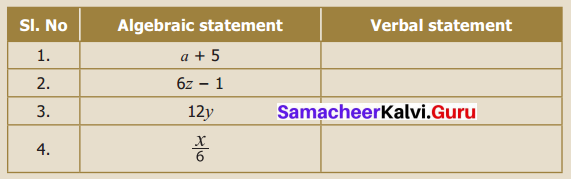Solution: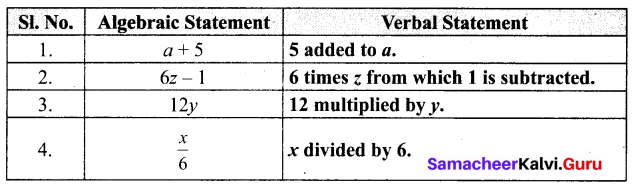Question 5.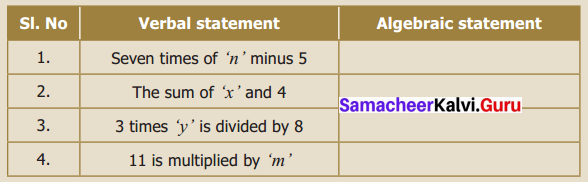Solution: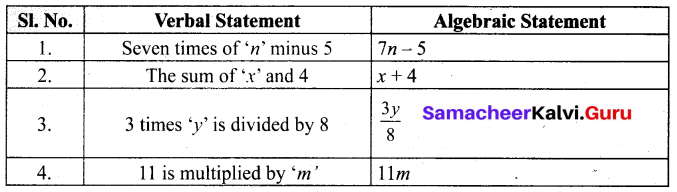Question 6.
Find the unknown.
(i) 37 + 43 = 43 + ____
(ii) (22 + 10) + 15 = ____ + (10 + 15)
(iii) If 7 × 46 = 322, then 46 × 7 = _____
Solution:
(i) 37 + 43 = 43 + 37
(ii) (22 + 10) + 15 = 22 + (10 + 15)
(iii) If 7 × 46 = 322, then 46 × 7 = 322

Question 7.
Find the suitable value of ‘m’, to get a sum of 9?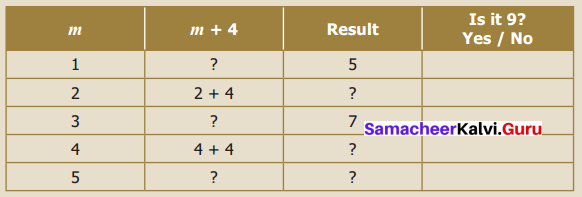Solution: